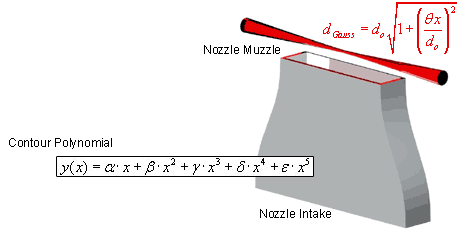# Multiphysics Displayed in Origin 7.5: Laser Synthesis of Nanoparticles

Dr. James Gardner
Environmental Instruments, Corp.

Summary

Dr. James Gardner, a consultant in nanotechnology and multiphysics engineering, has designed a nozzle to be used in the fabrication of integrated optics on ø100mm silicon wafers.Illustration of the nozzle/laser setup

A designed laminar flow nozzle issues reactants into a focused laser beam. The fluid and laser interpenetrate orthogonally. Critical nozzle geometry was derived using Computational Fluid Dynamics (CFD) code. To verify the computational model, Hot Wire Anemometry (HWA) was used to measure the fluid velocity profile. The goal was to design a nozzle that had a top-hat velocity profile (not the standard laminar parabolic) relative to the focused laser beam thickness.Making Presentation Quality Graphs Computational model, theory and experiment are shown in a single Origin scatter plot. The graph shows nozzle muzzle position versus normalized ordinate data. As the physical units of the ordinates are dissimilar, their values were divided by their respective maximum value to show the relative variance across the nozzle. The graph includes the following plots: Theoretical: The BLACK line is the Gaussian beam waist through a 60 inch focal length refractive optic. The equation noted in black in the bottom right corner of the graph is the result. The Origin nonlinear curve fitter was used to generate this curve. The ORANGE line is the Gaussian beam waist through an 18 inch focal length refractive optic. This was used as a comparison of off-the-shelf optics. Note the shorter focal length yields a shorter 'collimated' length (Rayleigh range).Computation:

1. The BLUE line is the Origin second-order polynomial curve fit to the locus of computational model prediction of velocity profile. The model generates a velocity vector every two millimeters. The vector magnitudes are then fit to the second-order polynomial. The equation that describes the blue curve fit line is also in blue labeled wCFD.

Experimental:

1. The RED Scatter plot is the actual HWA data of the muzzle velocity profile. HWA is a technique that immerses a thin wire in a flow stream. A current is applied to the wire thereby heating the wire. The current required to maintain the wire temperature is proportional to the convective cooling of the wire by the flow stream. This convective heat loss is directly proportional to the flow stream velocity. Each data point is the average value of 1,024 measurement (1Kbyte) taken at a 1KHz frequency. The error bars are the standard deviation of those 1,024 points. The legend shows this as the red diamonds labeled Hot Wire Data.

Again, Origin's polynomial curve fitting was used to generate the curve fit of the red data scatter plot shown as the RED line. If the model and experiment were in perfect agreement, the blue and red line would be coincident. In this case, model and experiment have a maximum deviation of approximately ten percent. The legend shows the curve fit as Hot Wire Data. The equation is shown as wHWA.

2. The GREEN Scatter Plot with error bars is a sanity check. A simple test was performed to check the Gaussian beam waist calculation. Polycarbonate blocks were placed on the nozzle muzzle at varying positions. The laser is then shuttered rapidly to create burned holes in the polycarbonate blocks. This procedure was performed eleven separate times at the plotted eleven positions. The errors bars are large due to the fact that the shutter was operated manually and the beam exposure time varied accordingly. This varying exposure time yielded inconsistent polycarbonate melting. After each experiment, the melted hole was measured with vernier calipers. We can see for the purposes of a sanity check the green data agrees with the black calculated curve.

3. Last, the diameter of a 100 mm silicon wafer is shown centered over the nozzle center. This shows the most uniform portions of the laser beam and reactant fluid are used for producing the most uniform nanoparticle coating.

Other Notes:Much use was made of Origin's extensive formatting utilities. Specifically, utilities for drawing lines, centerlines, dashed lines and Legend formatting was employed. The lines were created using the Line tool, activated by clicking the Line button on the Tools toolbar (2nd from the bottom left corner in the image seen here).

A vertical dashed/dotted green line was added to illustrate the axis symmetry. In an ideal situation, the left and right side of each data plot should be a mirror image. From inspection of the plot, the data are very close mirror images about the nozzle centerline.Origin's In-place text editing and Text Control dialog were used to customize the Legend.

MathType™ was used to display the equations.

Biography
Dr. James Gardner is a nanotechnology consultant. His work crosses into many areas including fluid mechanics, heat transference, chemical engineering, optical engineering, and mechanical design.

MathType™ is a trademark of Design Science